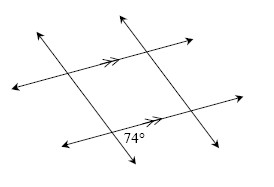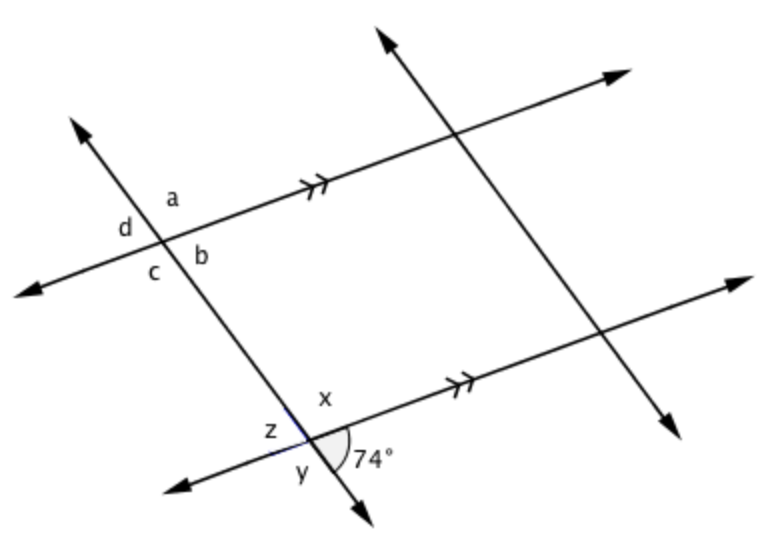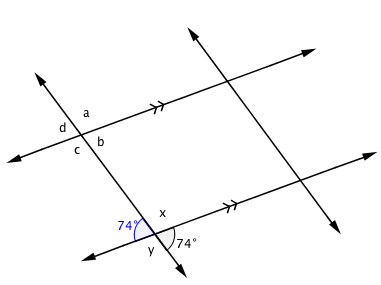### Home > GC > Chapter 2 > Lesson 2.3.2 > Problem2-111

2-111.

Examine the diagram below. Based on the information in the diagram, which angles can you determine? Copy the diagram on your paper and find only those angles that you can justify.Refer to the Math Notes box in Lesson 2.1.4.

The symbols on two of the lines indicate that those lines are parallel.

Since $z$ and the angle marked $74°$ are vertical angles, they are congruent.

The value of $x$ can be found because it is supplementary with both $74°$ angles.

Use clues from finding angle $z$ to find angle $y$. You can also find angles (a) through (d) since they correspond to known angles on parallel lines.